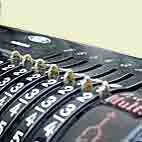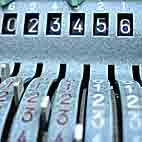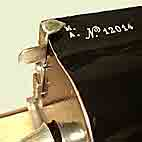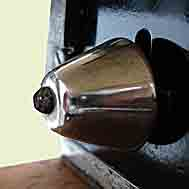Auxiliary attachments

Display

A security is brought to calculations if the input device is associated to a display to check if the correct number has been entered before starting the calculation (or after the calculation if the input device has not been restored). Some calculators with a full keyboard allow this check without display when the keys remain depressed.

Calculator without displayThe above photo is an example of calculator without input display. However, the entered number can be checked by watching the input levers positions. The input levers are not in a straight line when the digits of the number are different from each other and the checking is more difficult than in the case of a machine with a display.

Calculator with a displayAutomatic zeroing of the inscriptor

Most of calculators had a lever (or a key) to control the automatic clearance of the keyboard. When this lever is in +/- (or "A") position, the amount set on the keyboard automatically clears for each turn of the driving mechanism. This is not the case if the lever is set in the x/÷ ("M' or "R") position. One can find this zeroing lever on non keyboard input calculators. For example, on some Dactyle calculators, one of the first machines with pinwheels of Odhner manufactured in France.

Dactyle calculator with an automatic zeroing leverThe bell for the accumulator overflow (underflow)

Division is achieved by successive subtractions. In other words, if you want to calculate 22/7, you ask "how much time 7 is contained into 22".

The operation starts by registering the dividend 22 (in the leftmost part of the accumulator), and clearing the counter.

Divider 7 is then set on the input device just under the figure of the units of the dividend. Then the operator withdraws as many times as possible. The display of the counter shows the result of the division (number of subtractive cycles carried out). Then it is necessary that the operator makes sure before each subtractive cycle that the contents of the accumulator is greater or equal to divider 7.

When too many subtractions are done, the accumulator contains a negative number writen in its complementary form (starting with one or more 9). To cancel this, the operator turns the crank in the opposite direction as many times as necessary.

For the majority of manual calculators, a ring prevents the operator that one overturn has been done. A crank turn in the opposite direction (addition) cancels the preceding one (the bell rings again). The counter indicates 3 which is the whole part of division.

To pass to the first decimal, one shifts left the carriage of one position to start again the subtractions cycles exactly in the same way, and so on for the following digits. The result of the division is 3,14......, the remainder can be read on the accumulator dials.